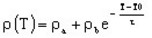# Resistivity, exponential function of T

## Presentation

This model defines a resistivity ρ as an exponential function of the temperature for an isotropic or anisotropic material.

## Mathematical model

The resistivity is an exponential function of the temperature.

The corresponding mathematical formula is written:where:

• T0 is the reference temperature [degree]
• ρa + ρb is the resistivity of the material at T0 [Ω .m]
• ρa is a constant quantity [Ω .m]
• τ is the temperature constant (positive or negative) [degree-1]

The shape of ρ(T) curve is presented in the figure below.## Anisotropic material

For an anisotropic material, the exponential model consists of a group of three exponentials.